# Optimal Transport between Copulas for Clustering Time Series

16 Mar 2016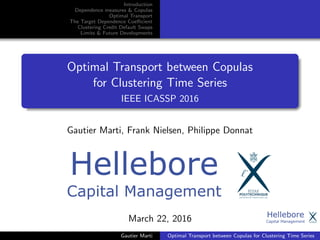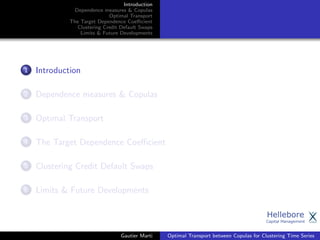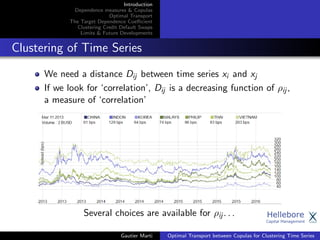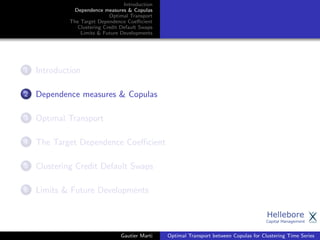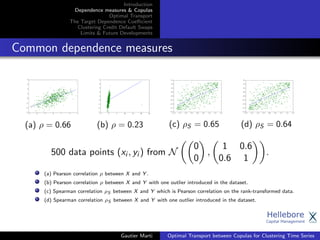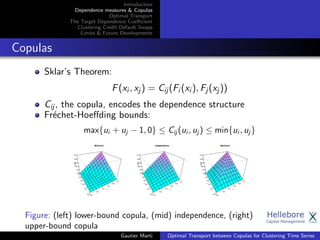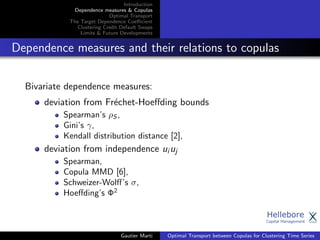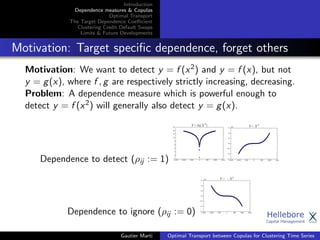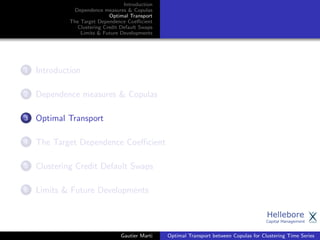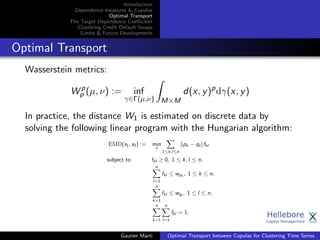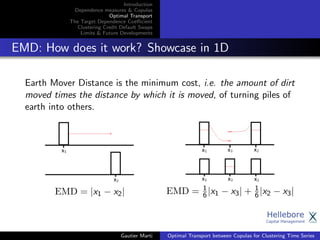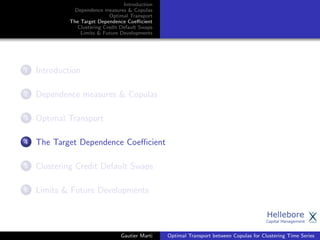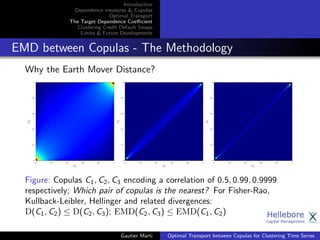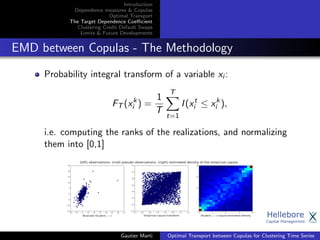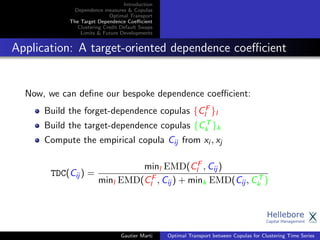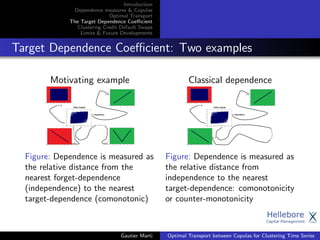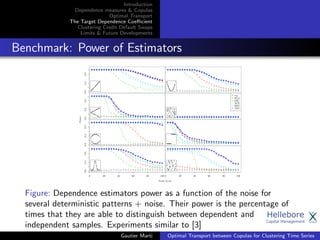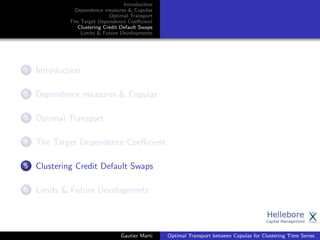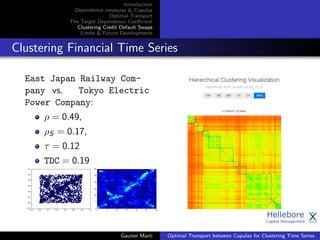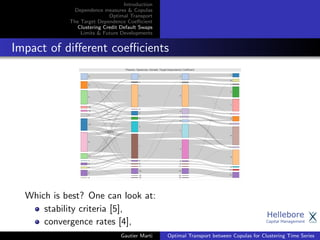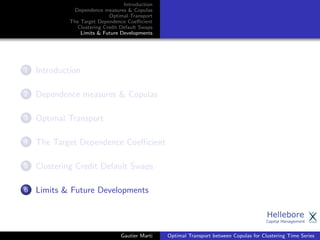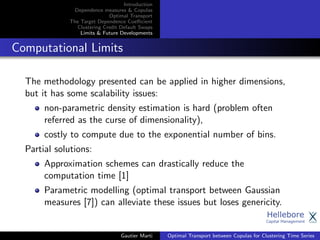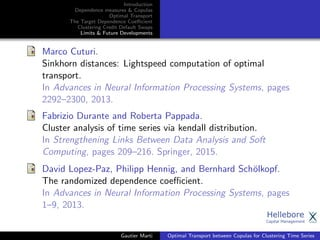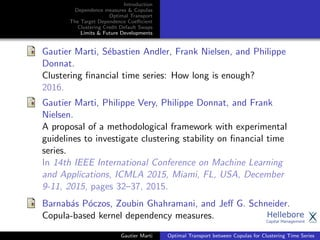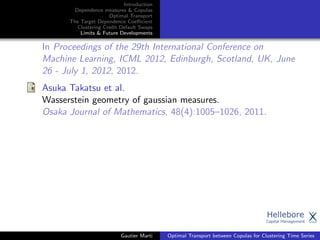1 sur 25

### Optimal Transport between Copulas for Clustering Time Series

• 1. Introduction Dependence measures & Copulas Optimal Transport The Target Dependence Coeﬃcient Clustering Credit Default Swaps Limits & Future Developments Optimal Transport between Copulas for Clustering Time Series IEEE ICASSP 2016 Gautier Marti, Frank Nielsen, Philippe Donnat March 22, 2016 Gautier Marti Optimal Transport between Copulas for Clustering Time Series
• 2. Introduction Dependence measures & Copulas Optimal Transport The Target Dependence Coeﬃcient Clustering Credit Default Swaps Limits & Future Developments 1 Introduction 2 Dependence measures & Copulas 3 Optimal Transport 4 The Target Dependence Coeﬃcient 5 Clustering Credit Default Swaps 6 Limits & Future Developments Gautier Marti Optimal Transport between Copulas for Clustering Time Series
• 3. Introduction Dependence measures & Copulas Optimal Transport The Target Dependence Coeﬃcient Clustering Credit Default Swaps Limits & Future Developments Clustering of Time Series We need a distance Dij between time series xi and xj If we look for ‘correlation’, Dij is a decreasing function of ρij , a measure of ‘correlation’ Several choices are available for ρij . . . Gautier Marti Optimal Transport between Copulas for Clustering Time Series
• 4. Introduction Dependence measures & Copulas Optimal Transport The Target Dependence Coeﬃcient Clustering Credit Default Swaps Limits & Future Developments 1 Introduction 2 Dependence measures & Copulas 3 Optimal Transport 4 The Target Dependence Coeﬃcient 5 Clustering Credit Default Swaps 6 Limits & Future Developments Gautier Marti Optimal Transport between Copulas for Clustering Time Series
• 5. Introduction Dependence measures & Copulas Optimal Transport The Target Dependence Coeﬃcient Clustering Credit Default Swaps Limits & Future Developments Common dependence measures (a) ρ = 0.66 (b) ρ = 0.23 (c) ρS = 0.65 (d) ρS = 0.64 500 data points (xi , yi ) from N 0 0 , 1 0.6 0.6 1 . (a) Pearson correlation ρ between X and Y . (b) Pearson correlation ρ between X and Y with one outlier introduced in the dataset. (c) Spearman correlation ρS between X and Y which is Pearson correlation on the rank-transformed data. (d) Spearman correlation ρS between X and Y with one outlier introduced in the dataset. Gautier Marti Optimal Transport between Copulas for Clustering Time Series
• 6. Introduction Dependence measures & Copulas Optimal Transport The Target Dependence Coeﬃcient Clustering Credit Default Swaps Limits & Future Developments Copulas Sklar’s Theorem: F(xi , xj ) = Cij (Fi (xi ), Fj (xj )) Cij , the copula, encodes the dependence structure Fr´echet-Hoeﬀding bounds: max{ui + uj − 1, 0} ≤ Cij (ui , uj ) ≤ min{ui , uj } Figure: (left) lower-bound copula, (mid) independence, (right) upper-bound copula Gautier Marti Optimal Transport between Copulas for Clustering Time Series
• 7. Introduction Dependence measures & Copulas Optimal Transport The Target Dependence Coeﬃcient Clustering Credit Default Swaps Limits & Future Developments Dependence measures and their relations to copulas Bivariate dependence measures: deviation from Fr´echet-Hoeﬀding bounds Spearman’s ρS , Gini’s γ, Kendall distribution distance , deviation from independence ui uj Spearman, Copula MMD , Schweizer-Wolﬀ’s σ, Hoeﬀding’s Φ2 Gautier Marti Optimal Transport between Copulas for Clustering Time Series
• 8. Introduction Dependence measures & Copulas Optimal Transport The Target Dependence Coeﬃcient Clustering Credit Default Swaps Limits & Future Developments Motivation: Target speciﬁc dependence, forget others Motivation: We want to detect y = f (x2) and y = f (x), but not y = g(x), where f , g are respectively strictly increasing, decreasing. Problem: A dependence measure which is powerful enough to detect y = f (x2) will generally also detect y = g(x). Dependence to detect (ρij := 1) Dependence to ignore (ρij := 0) Gautier Marti Optimal Transport between Copulas for Clustering Time Series
• 9. Introduction Dependence measures & Copulas Optimal Transport The Target Dependence Coeﬃcient Clustering Credit Default Swaps Limits & Future Developments 1 Introduction 2 Dependence measures & Copulas 3 Optimal Transport 4 The Target Dependence Coeﬃcient 5 Clustering Credit Default Swaps 6 Limits & Future Developments Gautier Marti Optimal Transport between Copulas for Clustering Time Series
• 10. Introduction Dependence measures & Copulas Optimal Transport The Target Dependence Coeﬃcient Clustering Credit Default Swaps Limits & Future Developments Optimal Transport Wasserstein metrics: W p p (µ, ν) := inf γ∈Γ(µ,ν) M×M d(x, y)p dγ(x, y) In practice, the distance W1 is estimated on discrete data by solving the following linear program with the Hungarian algorithm: EMD(s1, s2) := min f 1≤k,l≤n pk − ql fkl subject to fkl ≥ 0, 1 ≤ k, l ≤ n, n l=1 fkl ≤ wpk , 1 ≤ k ≤ n, n k=1 fkl ≤ wql , 1 ≤ l ≤ n, n k=1 n l=1 fkl = 1. Gautier Marti Optimal Transport between Copulas for Clustering Time Series
• 11. Introduction Dependence measures & Copulas Optimal Transport The Target Dependence Coeﬃcient Clustering Credit Default Swaps Limits & Future Developments EMD: How does it work? Showcase in 1D Earth Mover Distance is the minimum cost, i.e. the amount of dirt moved times the distance by which it is moved, of turning piles of earth into others. EMD = |x1 − x2| EMD = 1 6|x1 − x3| + 1 6|x2 − x3| Gautier Marti Optimal Transport between Copulas for Clustering Time Series
• 12. Introduction Dependence measures & Copulas Optimal Transport The Target Dependence Coeﬃcient Clustering Credit Default Swaps Limits & Future Developments 1 Introduction 2 Dependence measures & Copulas 3 Optimal Transport 4 The Target Dependence Coeﬃcient 5 Clustering Credit Default Swaps 6 Limits & Future Developments Gautier Marti Optimal Transport between Copulas for Clustering Time Series
• 13. Introduction Dependence measures & Copulas Optimal Transport The Target Dependence Coeﬃcient Clustering Credit Default Swaps Limits & Future Developments EMD between Copulas - The Methodology Why the Earth Mover Distance? Figure: Copulas C1, C2, C3 encoding a correlation of 0.5, 0.99, 0.9999 respectively; Which pair of copulas is the nearest? For Fisher-Rao, Kullback-Leibler, Hellinger and related divergences: D(C1, C2) ≤ D(C2, C3); EMD(C2, C3) ≤ EMD(C1, C2) Gautier Marti Optimal Transport between Copulas for Clustering Time Series
• 14. Introduction Dependence measures & Copulas Optimal Transport The Target Dependence Coeﬃcient Clustering Credit Default Swaps Limits & Future Developments EMD between Copulas - The Methodology Probability integral transform of a variable xi : FT (xk i ) = 1 T T t=1 I(xt i ≤ xk i ), i.e. computing the ranks of the realizations, and normalizing them into [0,1] Gautier Marti Optimal Transport between Copulas for Clustering Time Series
• 15. Introduction Dependence measures & Copulas Optimal Transport The Target Dependence Coeﬃcient Clustering Credit Default Swaps Limits & Future Developments Application: A target-oriented dependence coeﬃcient Now, we can deﬁne our bespoke dependence coeﬃcient: Build the forget-dependence copulas {CF l }l Build the target-dependence copulas {CT k }k Compute the empirical copula Cij from xi , xj TDC(Cij ) = minl EMD(CF l , Cij ) minl EMD(CF l , Cij ) + mink EMD(Cij , CT k ) Gautier Marti Optimal Transport between Copulas for Clustering Time Series
• 16. Introduction Dependence measures & Copulas Optimal Transport The Target Dependence Coeﬃcient Clustering Credit Default Swaps Limits & Future Developments Target Dependence Coeﬃcient: Two examples Motivating example Figure: Dependence is measured as the relative distance from the nearest forget-dependence (independence) to the nearest target-dependence (comonotonic) Classical dependence Figure: Dependence is measured as the relative distance from independence to the nearest target-dependence: comonotonicity or counter-monotonicity Gautier Marti Optimal Transport between Copulas for Clustering Time Series
• 17. Introduction Dependence measures & Copulas Optimal Transport The Target Dependence Coeﬃcient Clustering Credit Default Swaps Limits & Future Developments Benchmark: Power of Estimators 0.00.40.8 xvals power.cor[typ,] xvals power.cor[typ,] 0.00.40.8 xvals power.cor[typ,] xvals power.cor[typ,] cor dCor MIC ACE RDC TDC 0.00.40.8 xvals power.cor[typ,] xvals power.cor[typ,] 0 20 40 60 80 100 0.00.40.8 xvals power.cor[typ,] 0 20 40 60 80 100 xvals power.cor[typ,] Noise Level Power Figure: Dependence estimators power as a function of the noise for several deterministic patterns + noise. Their power is the percentage of times that they are able to distinguish between dependent and independent samples. Experiments similar to  Gautier Marti Optimal Transport between Copulas for Clustering Time Series
• 18. Introduction Dependence measures & Copulas Optimal Transport The Target Dependence Coeﬃcient Clustering Credit Default Swaps Limits & Future Developments 1 Introduction 2 Dependence measures & Copulas 3 Optimal Transport 4 The Target Dependence Coeﬃcient 5 Clustering Credit Default Swaps 6 Limits & Future Developments Gautier Marti Optimal Transport between Copulas for Clustering Time Series
• 19. Introduction Dependence measures & Copulas Optimal Transport The Target Dependence Coeﬃcient Clustering Credit Default Swaps Limits & Future Developments Clustering Financial Time Series East Japan Railway Com- pany vs. Tokyo Electric Power Company: ρ = 0.49, ρS = 0.17, τ = 0.12 TDC = 0.19 Gautier Marti Optimal Transport between Copulas for Clustering Time Series
• 20. Introduction Dependence measures & Copulas Optimal Transport The Target Dependence Coeﬃcient Clustering Credit Default Swaps Limits & Future Developments Impact of diﬀerent coeﬃcients Which is best? One can look at: stability criteria , convergence rates , Gautier Marti Optimal Transport between Copulas for Clustering Time Series
• 21. Introduction Dependence measures & Copulas Optimal Transport The Target Dependence Coeﬃcient Clustering Credit Default Swaps Limits & Future Developments 1 Introduction 2 Dependence measures & Copulas 3 Optimal Transport 4 The Target Dependence Coeﬃcient 5 Clustering Credit Default Swaps 6 Limits & Future Developments Gautier Marti Optimal Transport between Copulas for Clustering Time Series
• 22. Introduction Dependence measures & Copulas Optimal Transport The Target Dependence Coeﬃcient Clustering Credit Default Swaps Limits & Future Developments Computational Limits The methodology presented can be applied in higher dimensions, but it has some scalability issues: non-parametric density estimation is hard (problem often referred as the curse of dimensionality), costly to compute due to the exponential number of bins. Partial solutions: Approximation schemes can drastically reduce the computation time  Parametric modelling (optimal transport between Gaussian measures ) can alleviate these issues but loses genericity. Gautier Marti Optimal Transport between Copulas for Clustering Time Series
• 23. Introduction Dependence measures & Copulas Optimal Transport The Target Dependence Coeﬃcient Clustering Credit Default Swaps Limits & Future Developments Marco Cuturi. Sinkhorn distances: Lightspeed computation of optimal transport. In Advances in Neural Information Processing Systems, pages 2292–2300, 2013. Fabrizio Durante and Roberta Pappada. Cluster analysis of time series via kendall distribution. In Strengthening Links Between Data Analysis and Soft Computing, pages 209–216. Springer, 2015. David Lopez-Paz, Philipp Hennig, and Bernhard Sch¨olkopf. The randomized dependence coeﬃcient. In Advances in Neural Information Processing Systems, pages 1–9, 2013. Gautier Marti Optimal Transport between Copulas for Clustering Time Series
• 24. Introduction Dependence measures & Copulas Optimal Transport The Target Dependence Coeﬃcient Clustering Credit Default Swaps Limits & Future Developments Gautier Marti, S´ebastien Andler, Frank Nielsen, and Philippe Donnat. Clustering ﬁnancial time series: How long is enough? 2016. Gautier Marti, Philippe Very, Philippe Donnat, and Frank Nielsen. A proposal of a methodological framework with experimental guidelines to investigate clustering stability on ﬁnancial time series. In 14th IEEE International Conference on Machine Learning and Applications, ICMLA 2015, Miami, FL, USA, December 9-11, 2015, pages 32–37, 2015. Barnab´as P´oczos, Zoubin Ghahramani, and Jeﬀ G. Schneider. Copula-based kernel dependency measures. Gautier Marti Optimal Transport between Copulas for Clustering Time Series
• 25. Introduction Dependence measures & Copulas Optimal Transport The Target Dependence Coeﬃcient Clustering Credit Default Swaps Limits & Future Developments In Proceedings of the 29th International Conference on Machine Learning, ICML 2012, Edinburgh, Scotland, UK, June 26 - July 1, 2012, 2012. Asuka Takatsu et al. Wasserstein geometry of gaussian measures. Osaka Journal of Mathematics, 48(4):1005–1026, 2011. Gautier Marti Optimal Transport between Copulas for Clustering Time Series